# Numba, Pypy Overrated?

Many benchmarks show impressive performance gains with the use of Numba or Pypy. Numba allows to compile just-in-time some specific methods, while Pypy takes the approach of compiling/optimizing the full python program: you use it just like the standard python runtime. From those benchmarks, I imagined that those tools would improve my 2D Heston PDE solver performance easily. The initialization part of my program contains embedded for loops over several 10Ks elements. To my surprise, numba did not improve anything (and I had to isolate the code, as it would not work on 2D numpy arrays manipulations that are vectorized). I surmise it does not play well with scipy sparse matrices. Pypy did not behave better, the solver became actually slower than with the standard python interpreter, up to twice as slow, for example, in the case of the main solver loop which only does matrix multiplications and LU solves sparse systems. I did not necessarily expect any performance improvement in this specific loop, since it only consists in a few calls to expensive scipy calculations. But I did not expect a 2x performance drop either.

While I am sure that there are good use cases, especially for numba, I was a bit disappointed that it likely would require to significantly change my code to have any effect (possibly to not do the initialization with scipy sparse matrices, but then, it is not so clear how). Also, I find most benchmarks dumb. For example, the 2D ODE solver of the latter uses a simple dense 2D numpy array. On real code, things are not as simple.

Next I should try Julia again out of curiosity. I tried it several years ago in the context of a simple Monte-Carlo simulation and my experiment at the time was not all that encouraging, but it may be much better at building PDE solvers and not too much work to port my python code. I am curious to see if it ends up being faster, and whether the accessible LU solvers are any good. If Julia delivers, it’s a bit of a shame for python, since it has so many excellent high quality numerical libraries. Also when I look back at my old Monte-Carlo test, I bet it would benefit greatly from numba, somewhat paradoxically, compared to my current experiment.

# Fixing NaNs in Quadprog

Out of curiosity, I tried quadprog as open-source quadratic programming convex optimizer, as it is looks fast, and the code stays relatively simple. I however stumbled on cases where the algorithm would return NaNs even though my inputs seemed straighforward. Other libraries such as CVXOPT did not have any issues with those inputs.

Searching on the web, I found that I was not the only one to stumble on this kind of issue with quadprog. In particular, in 2014, Benjamen Tyner gave a simple example in R, where solve.QP returns NaNs while the input is very simple: an identity matrix with small perturbations out of the diagonal. Here is a copy of his example:

    library(quadprog)

n <- 66L

set.seed(6860)
X <- matrix(1e-20, n, n)
diag(X) <- 1
Dmat <- crossprod(X)
y <- seq_len(n)
dvec <- crossprod(X, y)

Amat <- diag(n)
bvec <- y + runif(n)

sol <- solve.QP(Dmat, dvec, Amat, bvec, meq = n)

print(sol$solution) # this gives all NaNs Other people stumbled on similar issues. In my specific case, I was able to debug the quadprog algorithm and find the root cause: two variables $$g_c$$ and $$g_s$$ can become very small, and their square becomes essentially zero, creating a division by zero. If, instead of computing $$\frac{g_s^2}{g_c^2}$$ we compute $$\left(\frac{g_s}{g_c}\right)^2$$, then the division by zero is avoided as the two variables are of the same order.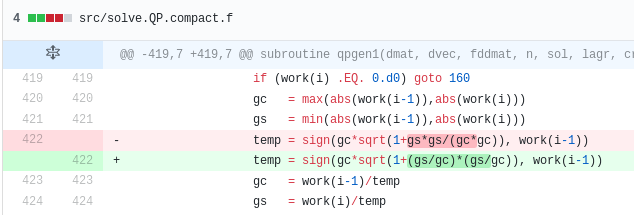Sample code change [on github](https://github.com/cran/quadprog/pull/1/commits/7f51915f7c662c7fac3d4e2ab067cfbc292767f8). While it probably does not catter for all the possible NaN use cases, it did fix all the cases I stumbled upon. # On the Probability of a Netflix Stock Crash This is a follow up to my previous post where I explore the probability of a TSLA stock crash, reproducing the results of Timothy Klassen. According to the implied cumulative probability density, TSLA has around 15% chance of crashing below$100. Is this really high compared to other stocks? or is it the interpretation of the data erroneous?

Here I take a look at NFLX (Netflix). Below is the implied volatility according to three different models.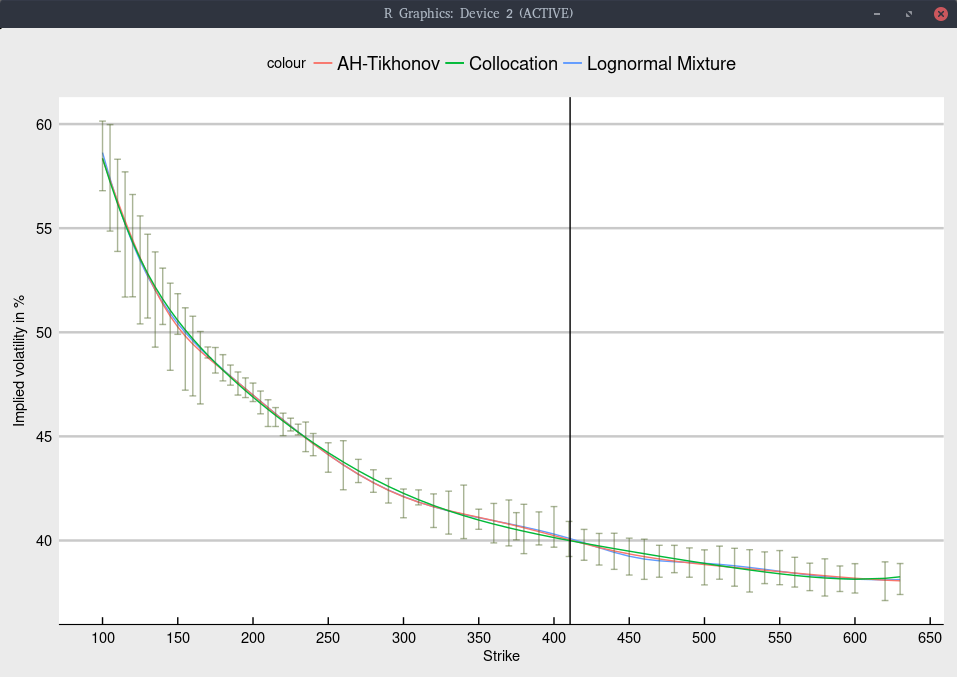Black volatility implied from Jan 2020 NFLX options.

Again, the shape is not too difficult to fit, and all models give nearly the same fit, within the bid-ask spread as long as we include an inverse relative bid-ask spread weighting in the calibration.

The corresponding implied cumulative density is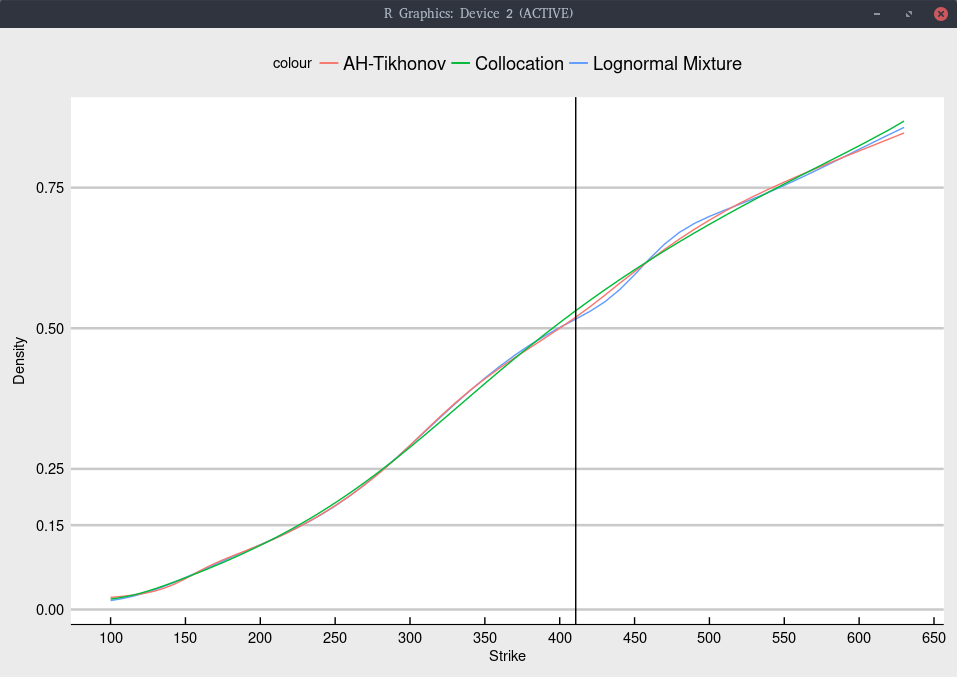Cumulative density implied from Jan 2020 NFLX options.

More explicitely, we obtain

Model probability of NFLX < 130
Collocation 3.3%
Lognormal Mixture 3.6%
Andreasen-Huge regularized 3.7%

The probability of NFLX moving below $130 is significantly smaller than the probability of TSLA moving below$100 (which is also around the same 13 of the spot price).

For completeness, the implied probability density is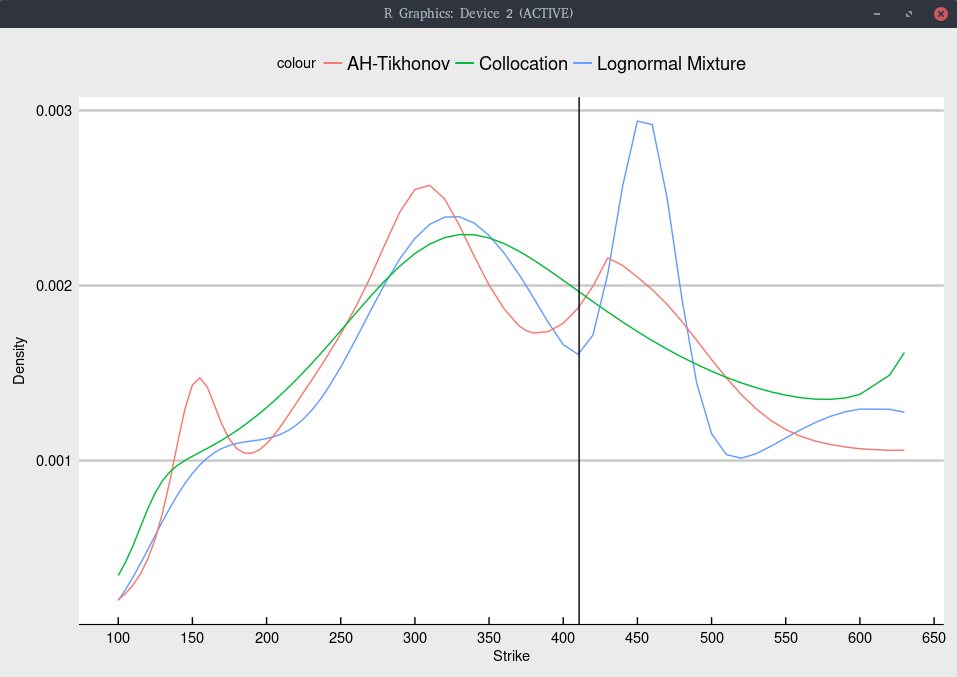Probability density implied from Jan 2020 NFLX options.

I did not particularly optimize the regularization constant here. The lognormal mixture can have a tendency to produce too large bumps in the density at specific strikes, suggesting that it could also benefit of some regularization.

What about risky stocks like SNAP (Snapchat)? It is more challenging to imply the density for SNAP as there are much fewer traded options on the NASDAQ: less strikes, and a lower volume. Below is an attempt.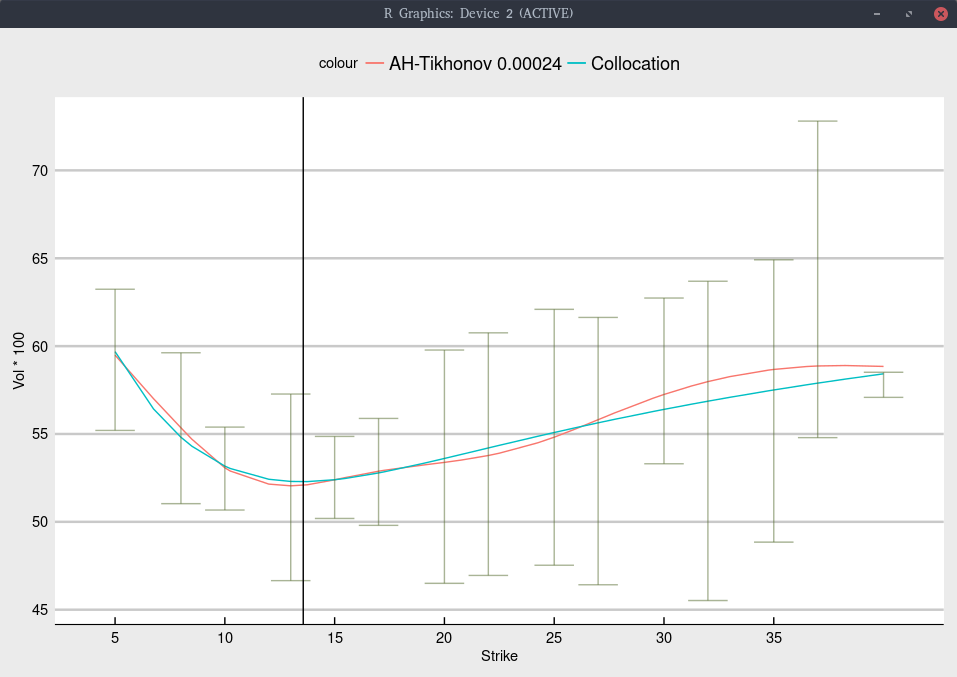Black volatility implied from Jan 2020 SNAP options.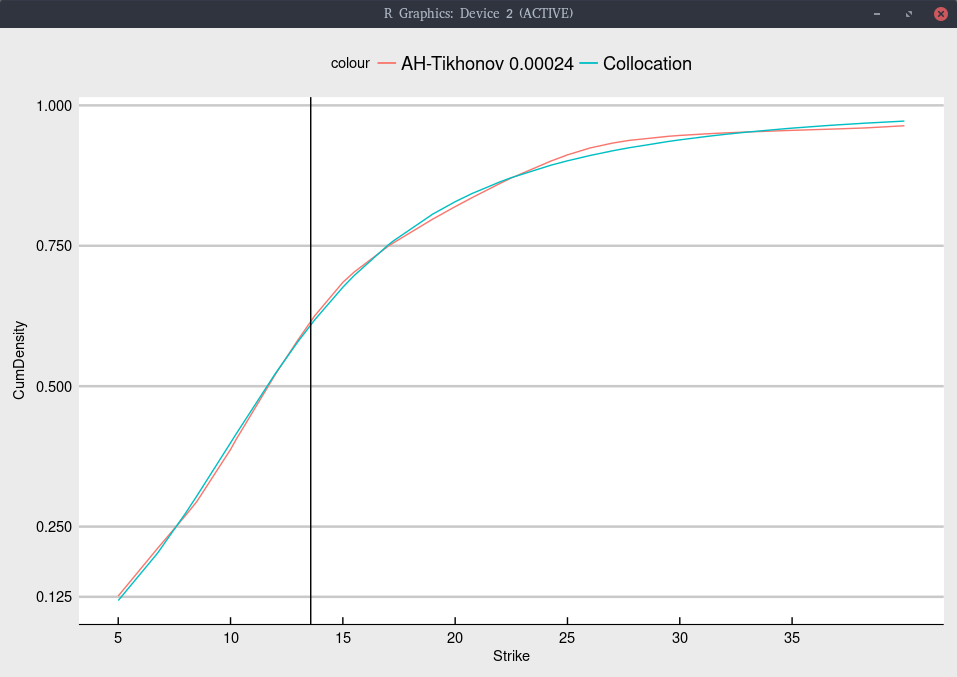Cumulative density implied from Jan 2020 SNAP options.

We find a probability of moving below 13 of the current price lower than with TSLA: around 12% SNAP vs 15% for TSLA.

# On the Probability of a TSLA Stock Crash

Timothy Klassen had an interesting post on linkedin recently, with the title “the options market thinks there is a 16% chance that Tesla will not exist in January 2020”. As I was also recently looking at the TSLA options, I was a bit intrigued. I looked at the option chain on July 10th, and implied the European volatility from the American option prices. I then fit a few of my favorite models: Andreasen-Huge with Tikhonov regularization, the lognormal mixture, and a polynomial collocation of degree 7. This results in the following graph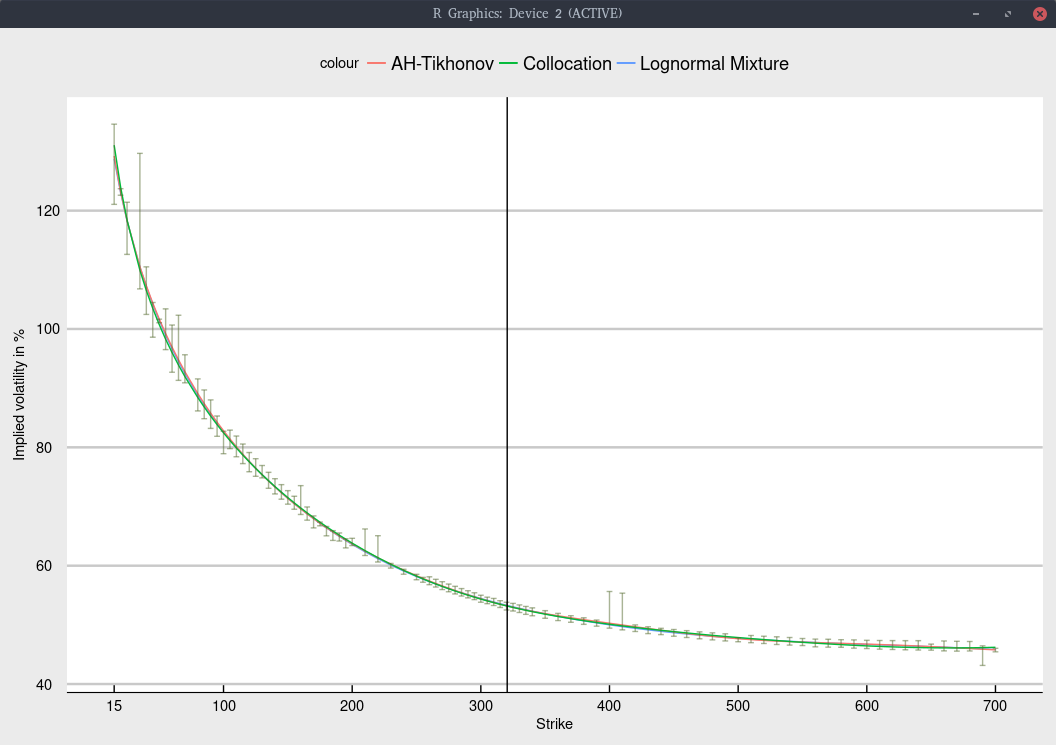Black volatility implied from Jan 2020 TSLA options.

The shape is not too difficult to fit, and all models give nearly the same fit, within the bid-ask spread as long as we include an inverse relative bid-ask spread weighting in the calibration. Note that I removed the quote for strike 30, as the bid-ask spread was, unusually extremely large and would only introduce noise.

Like Timothy Klassen, we can look at the implied cumulative density of each model.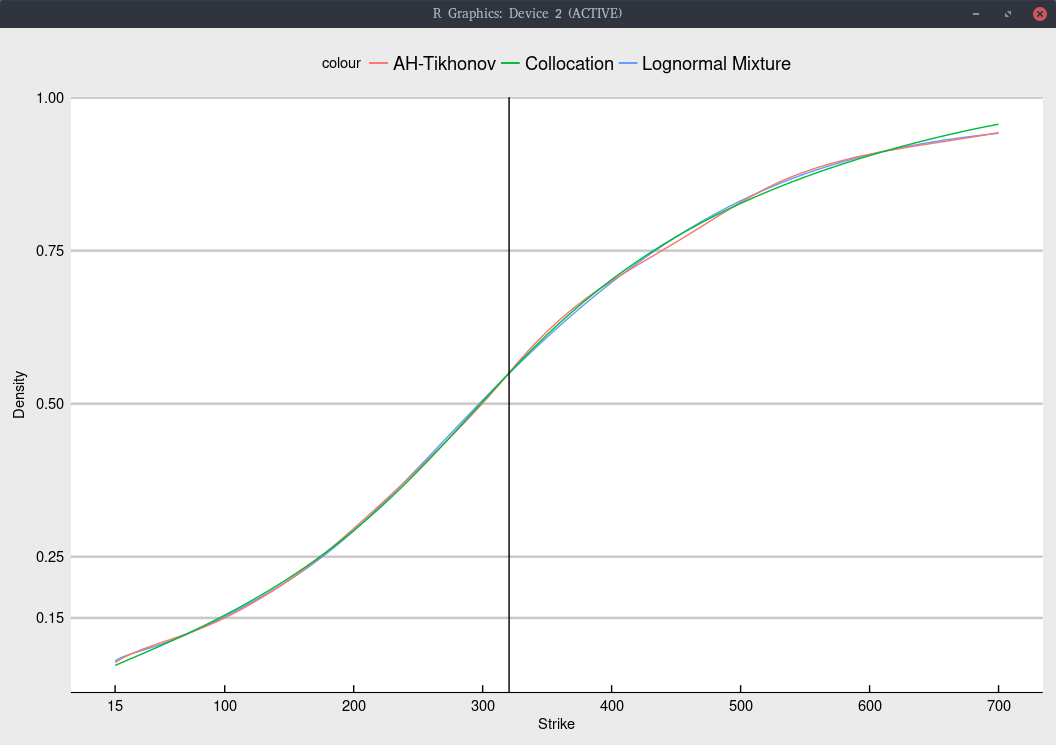Cumulative density implied from Jan 2020 TSLA options.

More explicitely, we obtain

Model probability of TSLA < 100 probability of TSLA < 15
Collocation 15.4% 7.2%
Lognormal Mixture 15.2% 8.0%
Andreasen-Huge regularized 15.0% 7.7%

It would have been great of Timothy Klassen had shown the implied density as well, in the spirit of my earlier posts on a similar subject.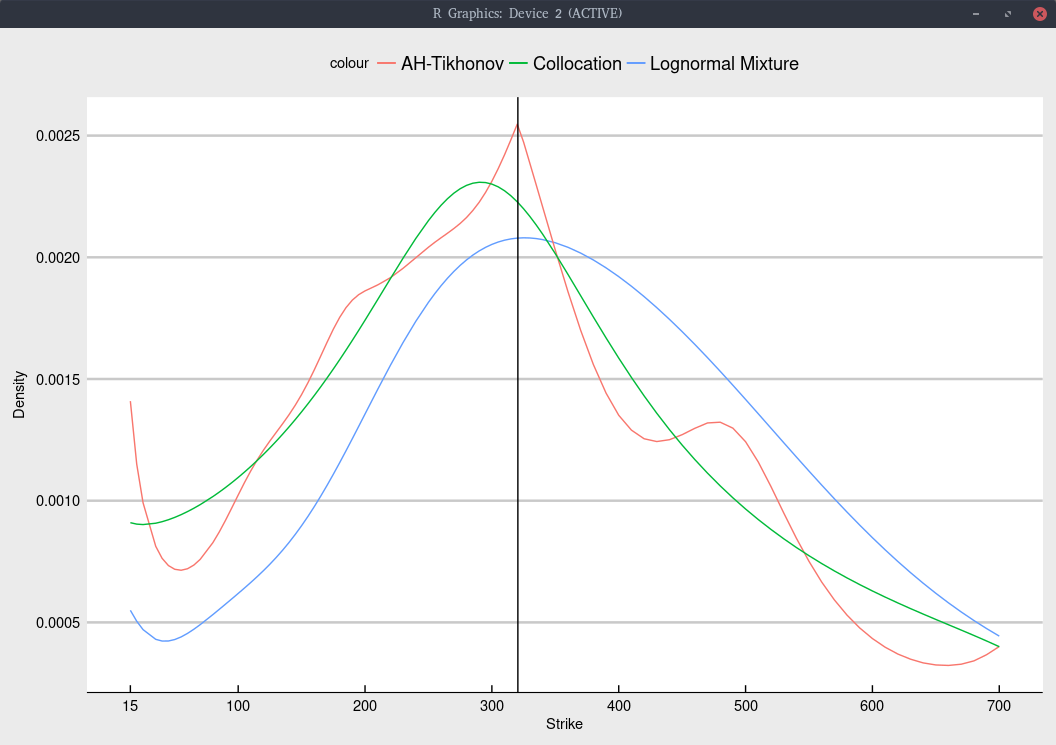Probability density implied from Jan 2020 TSLA options.

In particular, the choice of model has a much stronger impact on the implied density. Even though Andreasen-Huge has more modes, its fit in terms of volatilities is not better than the lognormal mixture model. We could reduce the number of modes by increasing the constant of the Tikhonov regularization, at the cost of a slightly worse fit.

The collocation produces a significantly simpler shape. This is not necessarily a drawback, since the fit is quite good and more importantly, it does not have a tendency to overfit (unlike the two other models considered).

Model weighted root mean square error in vols
Collocation 0.00484
Lognormal Mixture 0.00432
Andreasen-Huge regularized 0.00435

The most interesting from T. Klassen plot, is the comparison with the stock price across time. It is expected that the probability of going under $100 will be larger when the stock price moves down, which is what happen around March 27, 2018. But it is not so expected that when it comes back up higher (above$350 in mid June), the probability of going under $100 stays higher than it was before March 27, 2018, where the stock price was actually lower. In fact, there seems to be a signal in his time-serie, where the probability of going under$100 increases significantly, one week before March 27, that is one week before the actual drop, suggesting that the drop was priced in the options.

# The Fourth Moment of the Normal SABR Model

I was wondering if I could use the SABR moments to calibrate a model to SABR parameters directly. It turns out that the SABR moments have relatively simple expressions when $$\beta=0$$, that is, for the normal SABR model (with no absorption). This is for the pure SABR stochatic volatility model, not the Hagan approximation. For the Hagan approximation, we would need to use the replication by vanilla options to compute the moments.

I first saw a simple derivation of the fourth moment in Xavier Charvet and Yann Ticot 2011 paper Pricing with a smile: an approach using Normal Inverse Gaussian distributions with a SABR-like parameterisation. I discovered a more recent (2013) paper from Lorella Fatone et al. The use of statistical tests to calibrate the normal SABR model with simple formulae for the third and fourth moments (sci-hub.nu is your friend to read this). I had actually derived the third moment myself previously, based on the straighforward approach from Xavier Charvet, which consists merely in applying the Ito-Doeblin formula several times. Funnily, Xavier Charvet and Yann Ticot make it Irish, by calling it the Ito-Dublin formula.

Now the two papers lead to a different formula for the fourth moment. I did not have the courage to find out where exactly was the mistake of Lorella Fatone et al., as their paper is much more abstract, and follow a somewhat convoluted path to derive the formula. But I could not find a mistake in Xavier Charvet and Yann Ticot paper. They don’t directly give the fourth moment, but it is trivial to derive the centered fourth moment from their formulae. By centered, I mean the fourth moment of the process $$F(t)-F(0)$$ where $$F(0)$$ is the initial forward price.

$$\frac{A}{\nu^2}\left(e^{6\nu^2 t}-1\right)+2\frac{B}{\nu^2}\left(e^{3\nu^2 t}-1\right)+6\frac{C}{\nu^2}\left(e^{\nu^2 t}-1\right)$$ with $$A = \frac{\alpha^4 (1+ 4\rho^2)}{5 \nu^2}\,,$$ $$B = -\frac{2 \rho^2 \alpha^4}{\nu^2}\,,$$ $$C = - A - B \,.$$

Fatone et al. includes a factor $$\frac{1}{3}$$ in front of $$\rho^2$$, which I believe is a mistake. There is however no error in their third moment.

Unfortunately, my quick mapping idea does not appear to work so well for long maturities.

# Implying the Probability Density from Market Option Prices (Part 2)

This is a follow-up to my posts on the implied risk-neutral density (RND) of the SPW options before and after the big volatility change that happened in early February with two different techniques: a smoothing spline on the implied volatilities and a Gaussian kernel approach.

The Gaussian kernel (as well as to some extent the smoothing spline) let us believe that there are multiple modes in the distribution (multiple peaks in the density). In reality, Gaussian kernel approaches will, by construction, tend to exhibit such modes. It is not so obvious to know if those are real or artificial. There are other ways to apply the Gaussian kernel, for example by optimizing the nodes locations and the standard deviation of each Gaussian. The resulting density with those is very similar looking.

Following is the risk neutral density implied by nonic polynomial collocation out of the same quotes (Kees and I were looking at robust ways to apply the stochastic collocation):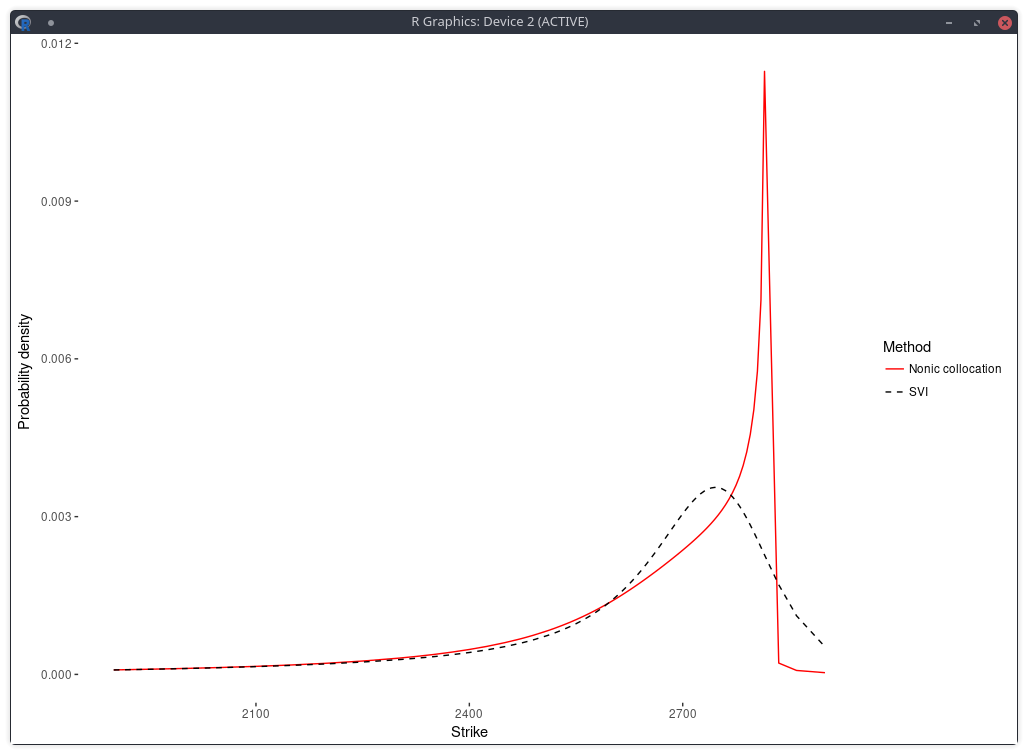probability density of the SPX implied from 1-month SPW options with stochastic collocation on a nonic polynomial.

There is now just one mode, and the fit in implied volatilities is much better.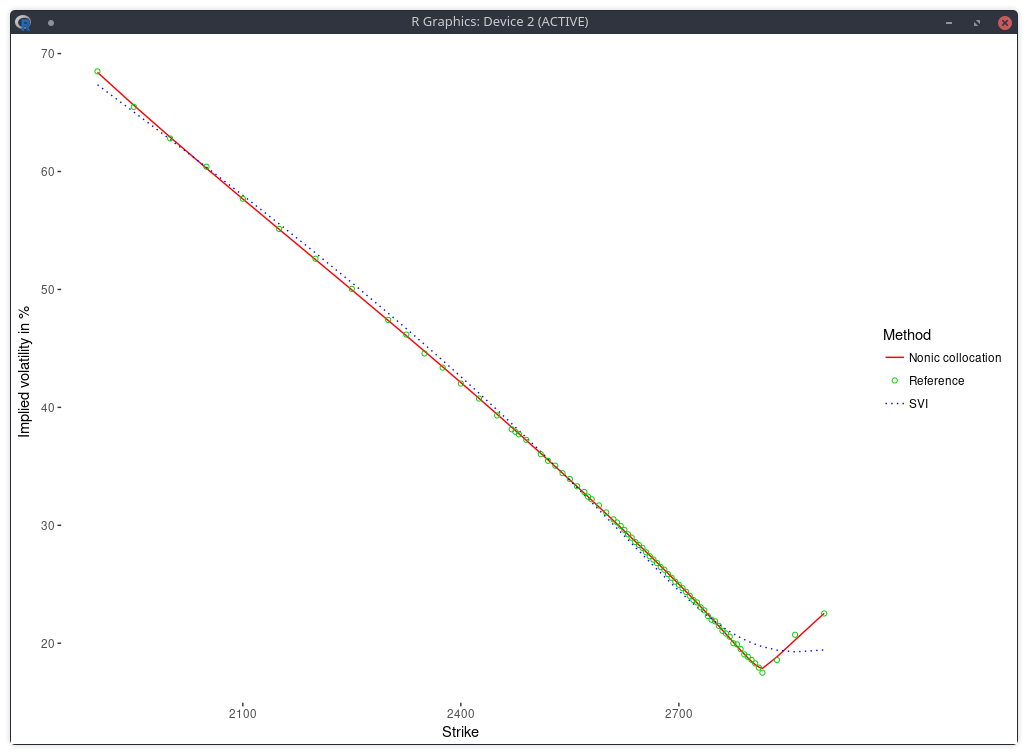implied volatility of the SPX implied from 1-month SPW options with stochastic collocation on a nonic polynomial.

In a related experiment, Jherek Healy showed that the Andreasen-Huge arbitrage-free single-step interpolation will lead to a noisy RND. Sebastian Schlenkrich uses a simple regularization to calibrate his own piecewise-linear local volatility approximation (a Lamperti-transform based approximation instead of the single step PDE approach of Andreasen-Huge). His Tikhonov regularization consists here in applying a roughness penalty consisting in the sum of squares of the consecutive local volatility slope differences. This is nearly the same as using the matrix of discrete second derivatives as Tikhonov matrix. The same idea can be found in cubic spline smoothing. This roughness penalty can be added in the calibration of the Andreasen-Huge piecewise-linear discrete local volatilities and we obtain then a smooth RND: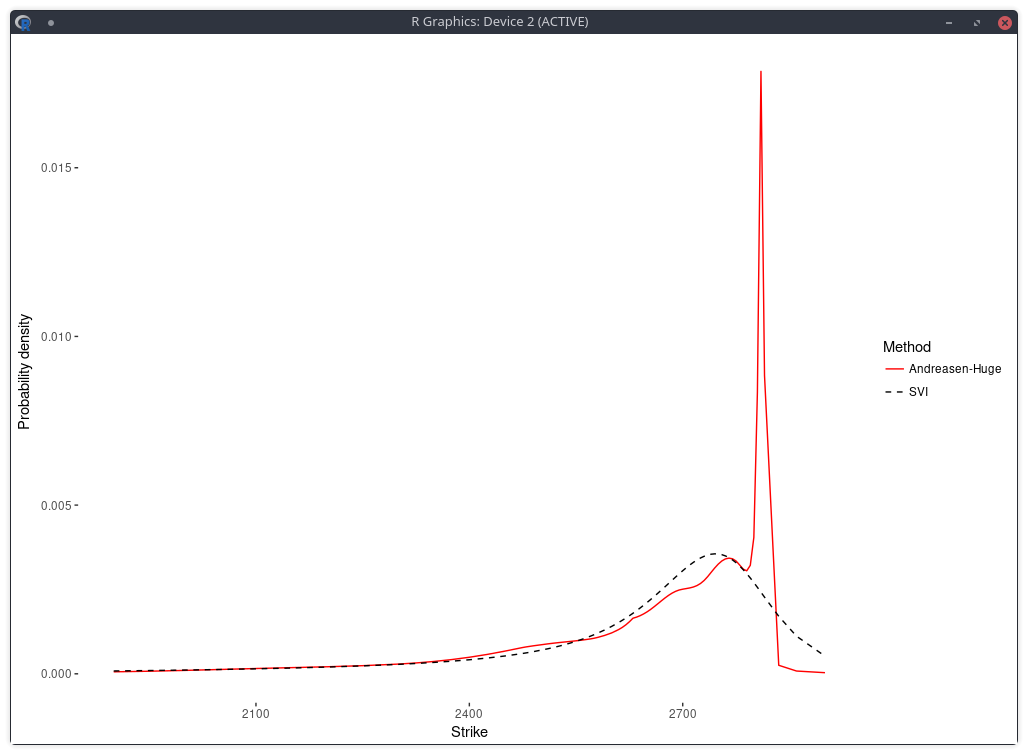density of the SPX implied from 1-month SPW options with Andreasen-Huge and Tikhonov regularization.

One difficulty however is to find the appropriate penalty factor $$\lambda$$. On this example, the optimal penalty factor can be guessed from the L-curve which consists in plotting the L2 norm of the objective against the L2 norm of the penalty term (without the factor lambda) in log-log scale, see for example this document. Below I plot a closely related function: the log of the penalty (with the lambda factor) divided by the log of the objective, against lambda. The point of highest curvature corresponds to the optimal penalty factor.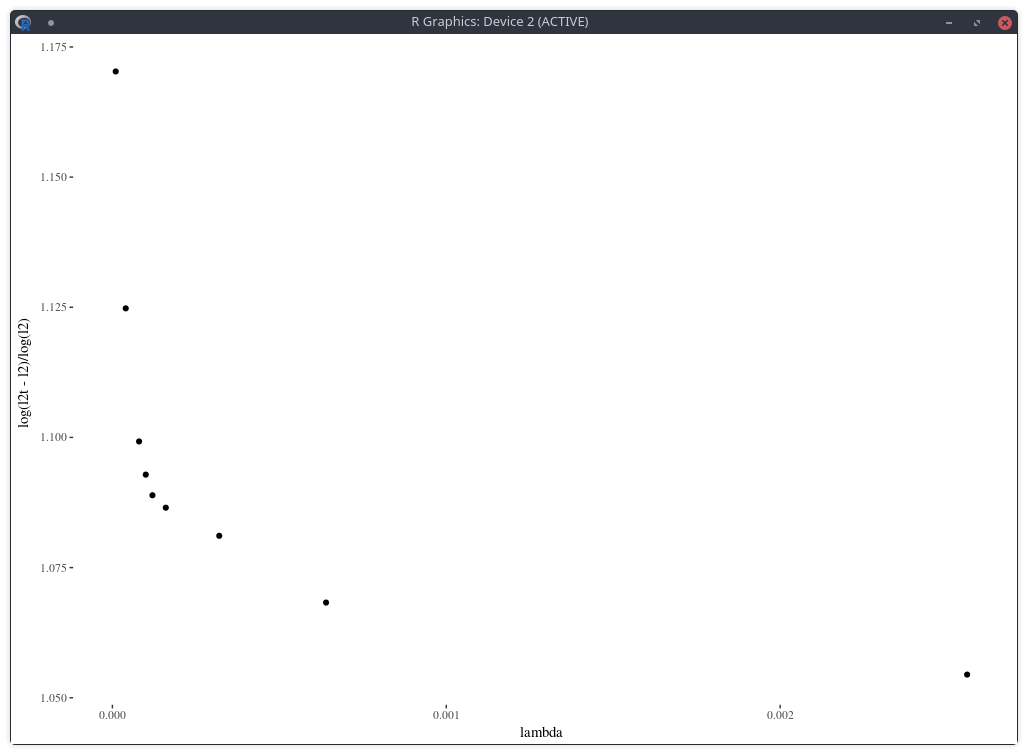density of the SPX implied from 1-month SPW options with nodes located at every 2 market strike.

Note that in practice, this requires multiple calibrations the model with different values of the penalty factor, which can be slow. Furthermore, from a risk perspective, it will also be challenging to deal with changes in the penalty factor.

The error of model versus market implied volatilies is similar to the nonic collocation (not better) even though the shape is less smooth and, a priori, less constrained as, on this example, the Andreasen-Huge method has 75 free-parameters while the nonic collocation has 9.

# Senior Developers Don't Know OO Anymore

It has been a while since the good old object-oriented (OO) programming is not trendy anymore. Functional programming or more dynamic programming (Python-based) have been the trend, with an excursion in template based programming for C++ guys. Those are not strict categories: Python can be used in a very OO way, but it’s not how it is marketed or considered by the community.

Recently, I have seen some of the ugliest refactoring in my life as a programmer, done by someone with at least 10 years of experience programming in Java. It is a good illustration because the piece of code is particularly simple (although I won’t bother with implementation details). The original code was a simple boolean method on an object such as

public class Dog
public boolean isHappy() { ... }
public void setHappy(boolean isHappy) { ... }

The methods were marked deprecated, and instead, new “utility” methods replaced it:

public class DogUtil
public static boolean isHappy(Dog dog) { ... }
public static void setHappy(Dog dog, boolean isHappy) { ... }

This is really breaking OO and moving back to procedural programming.

In a big (but not that big in reality) company, it is quite a challenge to avoid such code transformations. The code might be written by a team with a different manager, managers try to play nice to each other. And is it really worth fighting over such a trivial thing? if some low level (in the company hierarchy) programmer reports this, he is more likely to be labeled as a black sheep. Most managers prefer white sheeps.

More generally software is like entropy: it can start from a simple and clean state, but eventually it will always grow complex and somewhat ugly. And you end up with the Cobol syndrome, where people don’t really know anymore what the software is doing, can’t replace it as it is used (but nobody can tell exactly which parts), and “developers” do only small fixes and evolutions (patches) in an obsolete language on a system they don’t really understand.

# Implying the Probability Density from Market Option Prices

In the previous post, I showed a plot of the probability implied from SPW options before and after the big volatility change of last week. I created it from a least squares spline fit of the market mid implied volatilities (weighted by the inverse of the bid-ask spread). While it looks reasonable, the underlying technique is not very robust. It is particularly sensitive to the number of options strikes used as spline nodes.

Below I vary the number of nodes, by considering nodes at every N market strikes, for different values of N.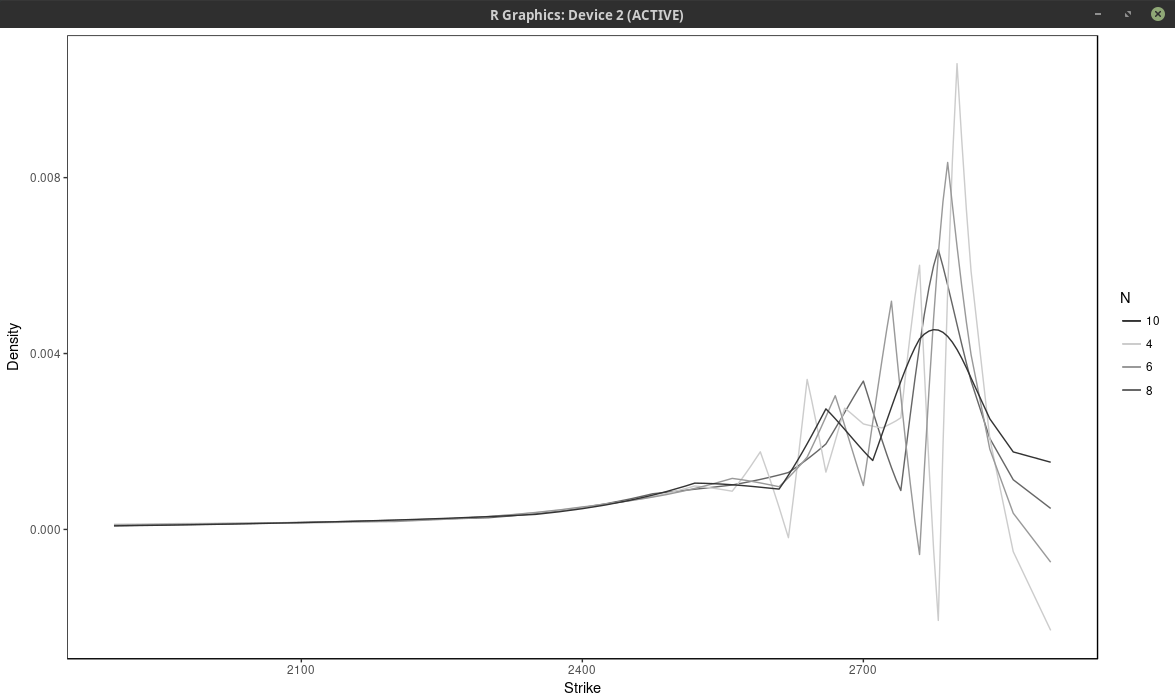probability density of the SPX implied from 1-month SPW options with nodes located at every N market strike, for different values of N. Least squares spline approach

With $$N \leq 6$$, the density has large wiggles, that go into the negative half-plane. As expected, a large N produces a flatter and smoother density. It is not obvious which value of N is the most appropriate.

Another technique is to fit directly a weighted sum of Gaussian densities, of fixed standard deviation, where the weights are calibrated to the market option prices. This is described in various papers and books from Wystup such as this one. He calls it the kernel slice approach. If we impose that the weights are positive and sum to one, the resulting model density will integrate to one and be positive.

It turns out that the price of a Vanilla option under this model is just the sum of vanilla options prices under the Bachelier model with shifted forwards (each “optionlet” forward corresponds to a peak on the market strike axis). So it is easy and fast to compute. But more importantly, the problem of finding the weights is linear. In deed, the typical measure to minimize is: $$\sum_{i=0}^{n} w_i^2 \left( C_i^M -\sum_{j=1}^m Q_j C_j^B(K_i) \right)^2$$ where $$w_i$$ is a market weight related to the bid-ask spread, $$C_i^M$$ is the market option price with strike $$K_i$$, $$C_j^B(K_i)$$ is the j-th Bachelier optionlet price with strike $$K_i$$ and $$Q_j$$ is the optionlet weight we want to optimize.

The minimum is located where the gradient is zero. $$\sum_{i=0}^{n} 2 w_i^2 C_k^B(K_i) \left( C_i^M -\sum_{j=1}^m Q_j C_j^B(K_i) \right) = 0 \text{ for } k=1,…,m$$ It is a linear and can be rewritten in term of matrices as $$A Q = B$$ but we have the additional constraints $$Q_j \geq 0$$ $$\sum_j Q_j = 1$$

The last constraint can be easily added with a Lagrange multiplier (or manually by elimination). The positivity constraint requires more work. As the problem is convex, the solution must lie either inside or on a boundary. So we need to explore each case where $$Q_k = 0$$ for one or several k in 1,…m. In total we have $$2^{m-1}-1$$ subsets to explore.

How to list all the subsets of $$\{1,…,m\}$$? It turns out it is very easy by using a bijection of each subset with the binary representation of $${0,…,2^m}$$. We then just need to increment a counter for 1 to $$2^m$$ and transform the binary representation to our original set elements. Each element of the subset corresponds to a 1 in the binary representation.

Now this works well if m is not too large as we need to solve $$2^{m-1}-1$$ linear systems. I actually found amazing, it took only a few minutes for m as high as 26 without any particular optimization. For m larger we need to be more clever. One possibility is to solve the unconstrained problem, and put all the negative quantities to 0 in the result, then solve again this new problem on those boundaries and repeat until we find a solution. This simple algorithm works often well, but not always. There exists specialized algorithms that are much better and nearly as fast. Unfortunately I was too lazy to code them. So I improvised on the Simplex. The problem can be transformed into something solvable by the Simplex algorithm. We maximize the function $$-\sum_j Z_j$$ with the constraints $$A Q - I Z = B$$ $$Q_j \geq 0$$ $$Z_j \geq 0$$ where I is the identity matrix. The additonal Z variables are slack variables, just here to help transform the problem. This is a trick I found on a researchgate forum. The two problems are not fully equivalent, but they are close enough that the Simplex solution is quite good.

With the spline, we minimize directly bid-ask weighted volatilities. With the kernel slice approach, the problem is linear only terms of call prices. We could use a non-linear solver with a good initial guess. Instead, I prefer to transform the weights so that the optimal solution on weighted prices is similar to the optimal solution on weighted volatilities. For this, we can just compare the gradients of the two problems: $$\sum_{i=0}^{n} 2 {w}_i^2 \frac{\partial C}{\partial \xi}(\xi, K_i) \left( C_i^M - C(\xi, K_i) \right)$$ with $$\sum_{i=0}^{n} 2 {w^\sigma_i}^2\frac{\partial \sigma}{\partial \xi}(\xi, K_i) \left( \sigma_i^M - \sigma(\xi, K_i)\right)$$ As we know that $$\frac{\partial C}{\partial \xi} = \frac{\partial \sigma}{\partial \xi} \frac{\partial C}{\partial \sigma}$$ we approximate $$\frac{\partial C}{\partial \sigma}$$ by the market Black-Scholes Vega and $$\left( C_i^M - C(\xi, K_i) \right)$$ by $$\frac{\partial C}{\partial \xi} (\xi_{opt}-\xi)$$, $$\left( \sigma_i^M - \sigma(\xi, K_i) \right)$$ by $$\frac{\partial \sigma}{\partial \xi} (\xi_{opt}-\xi)$$ to obtain $${w}_i \approx \frac{1}{ \frac{\partial C_i^M}{\partial \sigma_i^M} } {w^\sigma_i}$$

Now it turns out that the kernel slice approach is quite sensitive to the choice of nodes (strikes), but not as much as to the choice of number of nodes. Below is the same plot as with the spline approach, that is we choose every N market strike as node. For N=4, the density is composed of the sum of m/4 Gaussian densities. We optimized the kernel bandwidth (here the standard deviation of each Gaussian density), and found that it was relatively insensitive to the number of nodes, in our case around 33.0 (in general it is expected to be about three times the order the distance between two consecutive strikes, which is 5 to 10 in our case), a smaller value will translate to narrower peaks.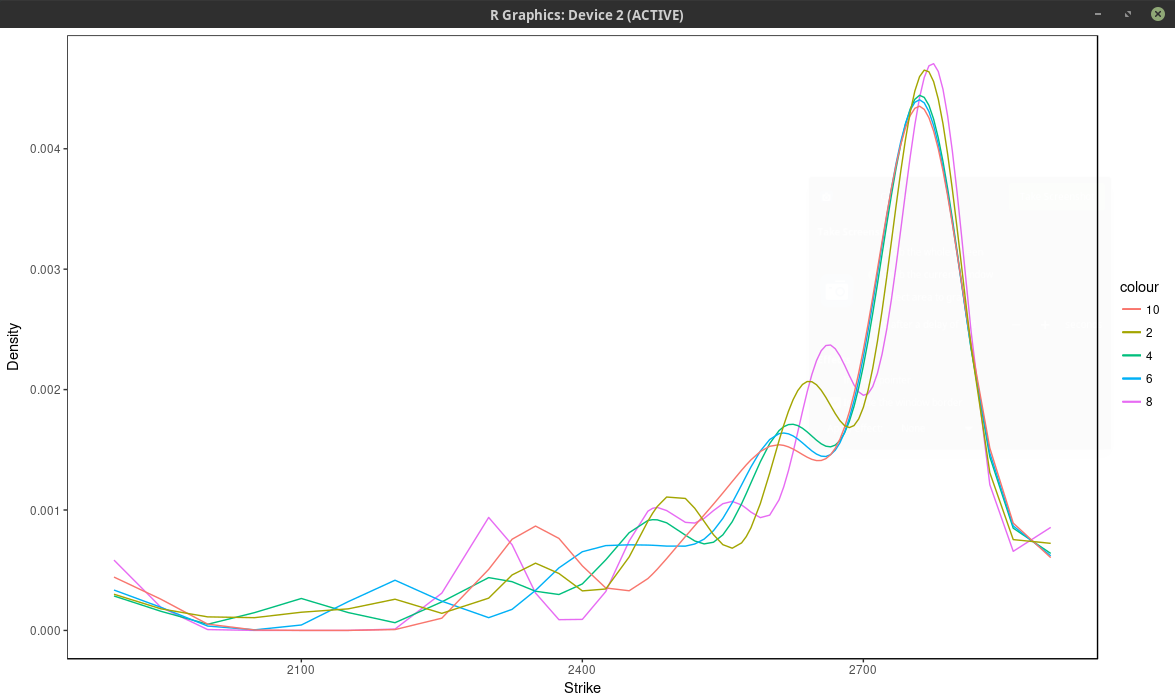probability density of the SPX implied from 1-month SPW options with nodes located at every N market strike, for different values of N. Kernel slice approach.

Even if we consider here more than 37 nodes (m=75 market strikes), the optimal solution actually use only 13 nodes, as all the other nodes have a calibrated weight of 0. The fit can be much better by adding nodes at f * 0.5, f * 0.8, f * 0.85, f * 0.9, f * 0.95, f * 0.98, f, f * 1.02, f * 1.05, f * 1.1, f * 1.2, f * 1.5, where f is the forward price, even though the optimal solution will only use 13 nodes again. We can see this by looking at the implied volatility.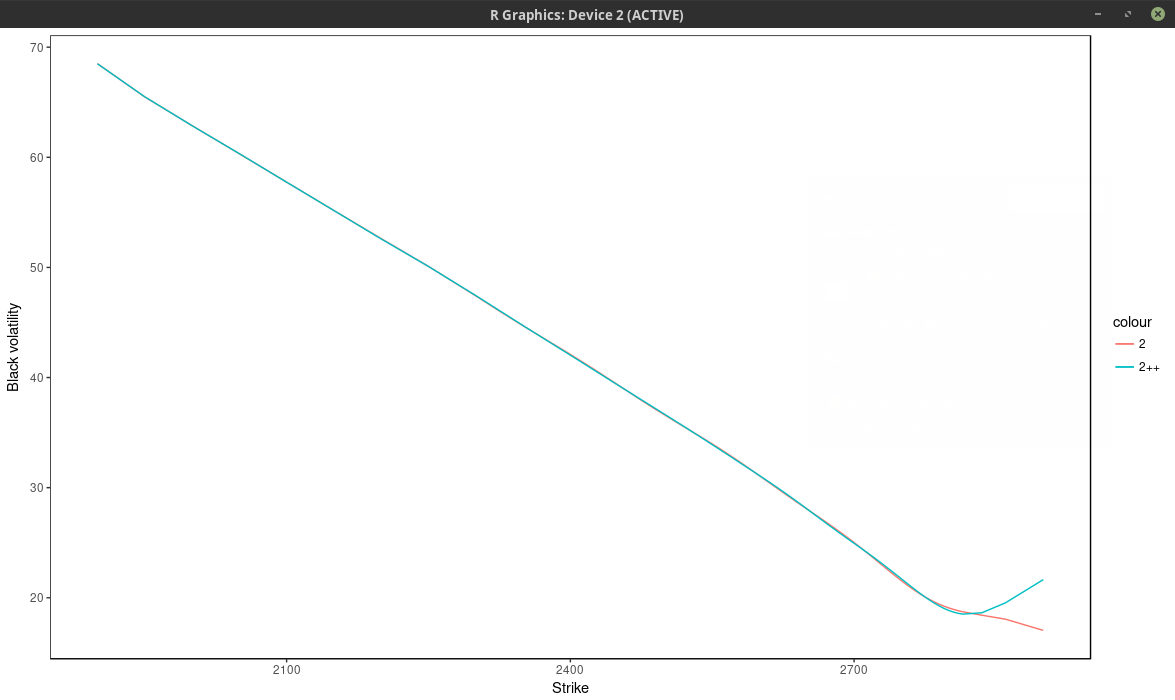implied volatility of the SPX implied from 1-month SPW options with nodes located at every 2 market strike.

Using only the market nodes does not allow to capture right wing of the smile. The density is quite different between the two.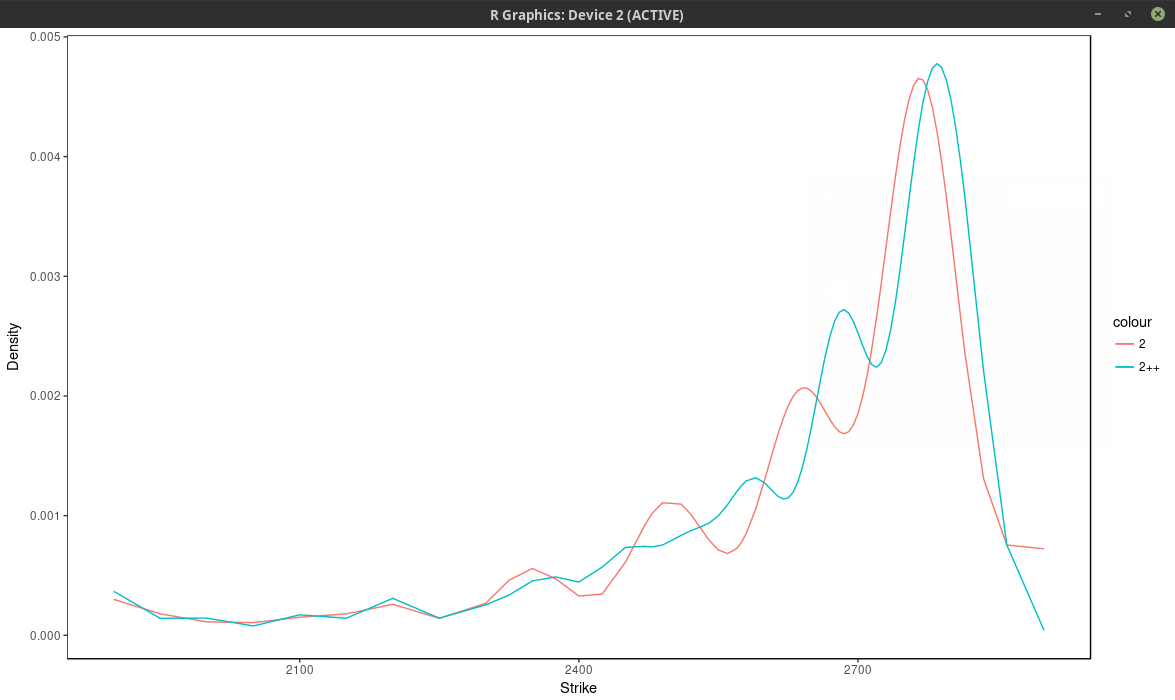density of the SPX implied from 1-month SPW options with nodes located at every 2 market strike.

I found (surprisingly) that even those specific nodes by themselves (without any market strike) work better than using all market strikes (without those nodes), but then we impose where the peaks will be located eventually.

It is interesting to compare the graph with the one before the volatility jump: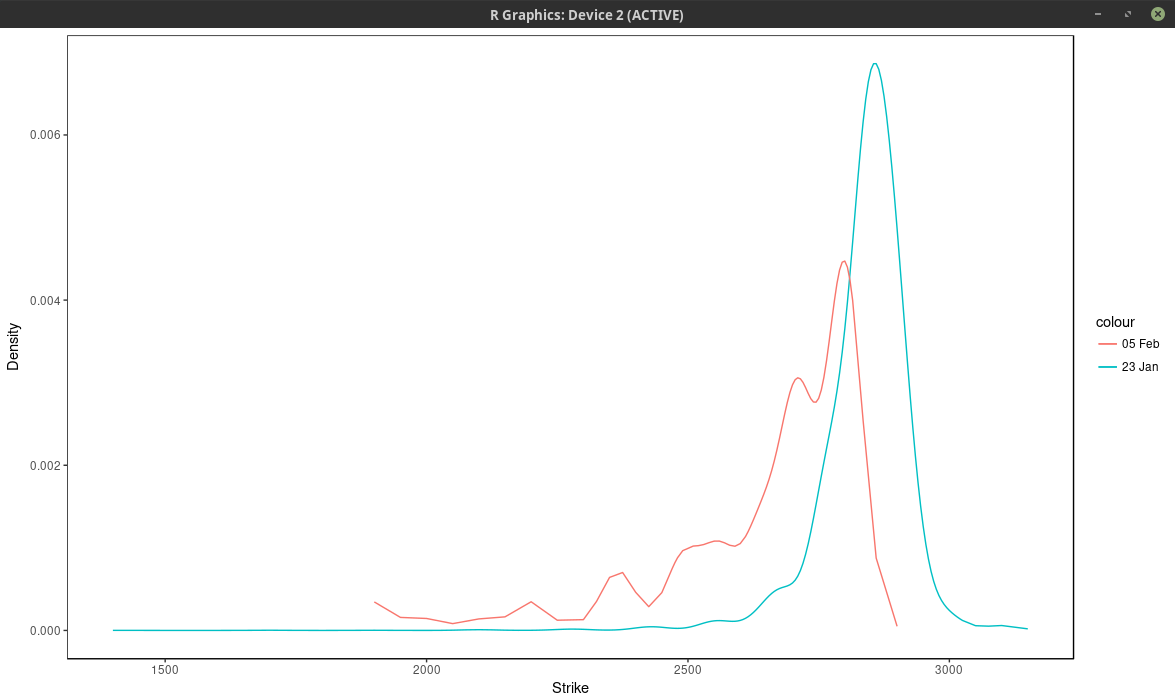density of the SPX implied from 1-month SPW options with nodes located at every 2 market strike.

So in calm markets, the density is much smoother and has really only one main mode/peak.

It is possible to use other kernels than the Gaussian kernel. The problem to solve would be exactly the same. It is not clear what would be the advantages of another kernel, except, possibly, speed to solve the linear system in O(n) operations for a compact kernel spanning at most 3 nodes (which would translate to a tridiagonal system).

# Where is the S&P 500 going to end?

Yesterday the American stocks went a bit crazy along with the VIX that jumped from 17.50 to 38. It’s not exactly clear why, the news mention that the Fed might raise its interest rates, the bonds yield have been recently increasing substantially, and the market self-correcting after stocks grew steadily for months in a low VIX environment.

I don’t exactly follow the SPX/SPW options daily. But I had taken a snapshot two weeks ago when the market was quiet. We can imply the probability density from the market option prices. It’s not an exact science. Here I do this with a least-squares spline on the implied volatilities (the least squares smoothes out the noise). I will show another approach in a subsequent post.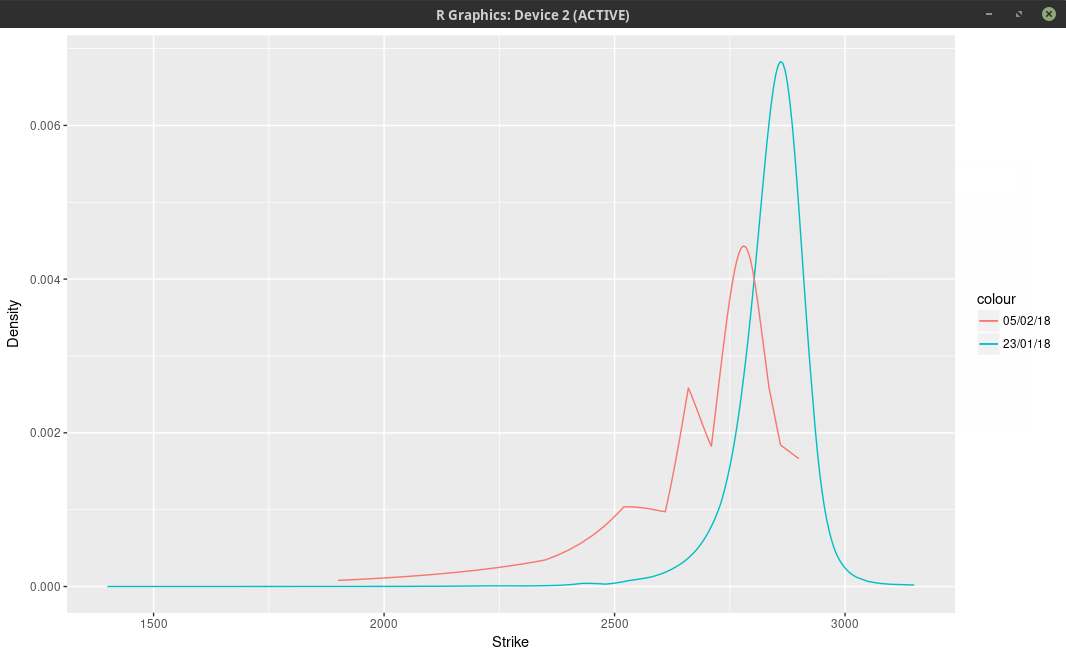probability density of the SPX implied from 1-month SPW options.

We can clearly see two bumps and a smaller third one further away in the left tail. This means that the market participants expect the SPX500 to go mostly to 2780 (slightly below where the spx future used to be) one month from now. Some market participants are more cautious and believe it could hover around 2660. Finally a few pessimists think more of 2530.

Option traders like to look at the implied volatilities (below).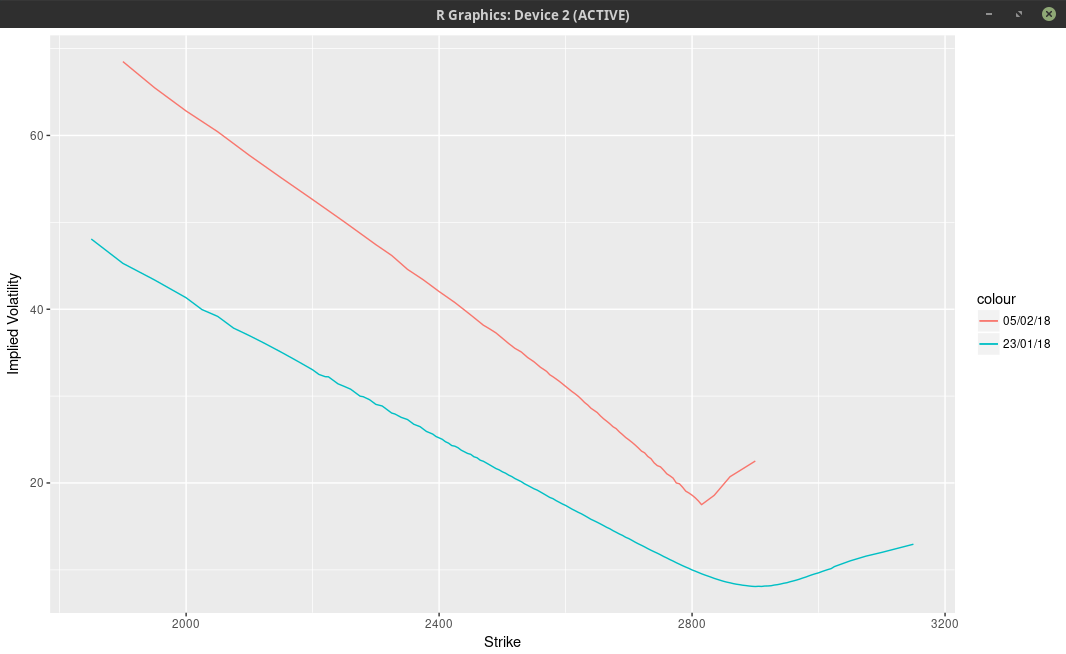implied volatilities of 1-month SPW options.

On the graph above, it’s really not clear that there is some sort of trimodal distribution. There is however an extremely sharp edge, that we normally see only for much shorter maturities. The vols are interpolated with a cubic spline above, not with a least-squares spline, in order to show the edge more clearly.

This is a bit reminiscent of the Brexit bets where Iain Clark shows two modes in the probability density of the GBP/USD.

# Discrete Sine Transform via the FFT

Several months ago, I had a quick look at a recent paper describing how to use Wavelets to price options under stochastic volatility models with a known characteristic function. The more classic method is to use some numerical quadrature directly on the Fourier integral as described in this paper for example. When I read the paper, I was skeptical about the Wavelet approach, since it looked complicated, and with many additional parameters.

I recently took a closer look and it turns out my judgment was bad. The additional parameters can be easily automatically and reliably set by very simple rules that work well (a subsequent paper by the same author, which applies the method to Bermudan options, clarifies this). The method is also not as complex as I first imagined. And more importantly, the FFT makes it fast. It is quite amazing to see the power of the FFT in action. It really is because of the FFT that the Shannon Wavelet method is practical.

Anyway one of the things that need to be computed are the payoff coefficients, and one expression is just the sum of a discrete Cosine transform (DCT) and a discrete Sine transform (DST). I was wondering then about a simple way to use the FFT for the Sine transform. There are many papers around how to use the FFT to compute the Cosine transform. A technique that is efficient and simple is the one of Makhoul.

The coefficients that need to be computed for all k can be represented by the following equation $$V_{k} = \sum_{j=0}^{N-1} a_j \cos\left(\pi k\frac{j+\frac{1}{2}}{N}\right) + b_j \sin\left(\pi k\frac{j+\frac{1}{2}}{N}\right)$$ with $$N= 2^{\bar{J}-1}$$ for some positive integer $$\bar{J}$$. Makhoul algorithm to compute the DCT of size N with one FFT of size N consists in

• initialize the FFT coefficients $$c_j$$ with: $$c_j = a_{2j} \quad\,,\quad c_{N-1-j} = a_{2j+1} \quad \text{ for } j = 0,…, \frac{N}{2}-1$$
• and then from the result of the FFT $$hat{c}$$, the DCT coefficients $$\hat{a}$$ are $$\hat{a}_k = \Re\left[ \hat{c}_j e^{-i \pi\frac{k}{2N}} \right]\,.$$

Makhoul does not specify the equivalent formula for the DST, but we can do something similar.

• We first initialize the FFT coefficients $$c_j$$ with: $$c_j = b_{2j} \quad\,,\quad c_{N-1-j} = -b_{2j+1} \quad \text{ for } j = 0,…, \frac{N}{2}-1$$
• and then from the result of the FFT $$\hat{c}$$, the DST coefficients $$\hat{b}$$ are $$\hat{b}_k = -\Im\left[ \hat{c}_j e^{-i \pi\frac{k}{2N}} \right]\,.$$

For maximum performance, the two FFTs can reuse the same sine and cosine tables. And the last step of the DCT and DST can be combined together.

Another approach would be to compute a single FFT of size 2N as we can rewrite the coefficients as $$V_{k} = \Re\left[e^{-i \pi \frac{k}{2N}} \sum_{j=0}^{2N-1} (a_j + i b_j) e^{-2i\pi k\frac{j}{2N}} \right]$$ with $$a_j = b_j =0$$ for $$j \geq N$$

In fact the two are almost equivalent, since a FFT of size 2N is decomposed internally into two FFTs of size N.

With this in mind we can improve a bit the above to merge the two transforms together:

• We first initialize the FFT coefficients $$c_j$$ with: $$c_j = a_{2j} + i b_{2j} \quad\,,\quad c_{N-1-j} = a_{2j+1} -i b_{2j+1} \quad \text{ for } j = 0,…, \frac{N}{2}-1$$
• and then from the result of the FFT $$\hat{c}$$, the coefficients are $$V_k = \Re\left[ \hat{c}_j e^{-i \pi\frac{k}{2N}} \right]\,.$$

And we have computed the coefficients with a single FFT of size N.

Submit to reddit
 © 2006-16 FabienThis work is licensed under a Creative Commons Attribution 4.0 International License.